The SEVERITY Procedure

Example 23.7 Defining a Finite Mixture Model That Has a Scale Parameter

A finite mixture model is a stochastic model that postulates that the probability distribution of the data generation process is a mixture of a finite number of probability distributions. For example, when an insurance company analyzes loss data from multiple policies that are underwritten in different geographic regions, some regions might behave similarly, but the distribution that governs some regions might be different from the distribution that governs other regions. Further, it might not be known which regions behave similarly. Also, the larger amounts of losses might follow a different stochastic process from the stochastic process that governs the smaller amounts of losses. It helps to model all policies together in order to pool the data together and exploit any commonalities among the regions, and the use of a finite mixture model can help capture the differences in distributions across regions and ranges of loss amounts.

Formally, if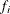and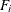denote the PDF and CDF, respectively, of component distribution i and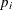represents the mixing probability that is associated with component i, then the PDF and CDF of the finite mixture of K distribution components are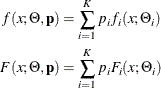where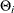denotes the parameters of component distribution i and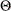denotes the parameters of the mixture distribution, which is a union of all theparameters.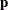denotes the set of mixing probabilities. All mixing probabilities must add up to 1 (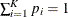).

You can define the finite mixture of a specific number of components and specific distributions for each of the components by defining the FCMP functions for the PDF and CDF. However, in general, it is not possible to fit a scale regression model by using any finite mixture distribution unless you take special care to ensure that the mixture distribution has a scale parameter. This example provides a formulation of a two-component finite mixture model that has a scale parameter.

To start with, each component distribution must have either a scale parameter or a log-transformed scale parameter. Let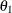and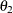denote the scale parameters of the first and second components, respectively. Let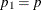be the mixing probability, which makes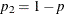by using the constraint on. The PDF of the mixture of these two distributions can be written as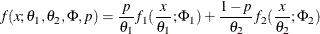where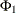and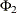denote the sets of nonscale parameters of the first and second components, respectively, and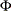denotes a union ofand. For the mixture to have the scale parameter, the PDF must be of the form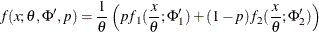where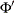,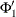, and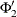denote the modified sets of nonscale parameters. One simple way to achieve this is to make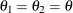and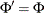; that is, you simply equate the scale parameters of both components and keep the set of nonscale parameters unchanged. However, forcing the scale parameters to be equal in both components is restrictive, because the mixture cannot model potential differences in the scales of the two components. A better approach is to tie the scale parameters of the two components by a ratio such that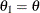and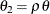. If the ratio parameter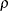is estimated along with the other parameters, then the mixture distribution becomes flexible enough to model the variations across the scale parameters of individual components.

To summarize, the PDF and CDF are of the following form for the two-component mixture that has a scale parameter: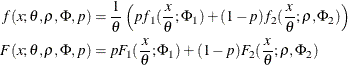This can be generalized to a mixture of K components by introducing the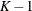ratio parameters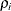that relate the scale parameters of each of the K components to the scale parameterof the mixture distribution as follows: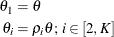In order to illustrate this approach, define a mixture of two lognormal distributions by using the following PDF function: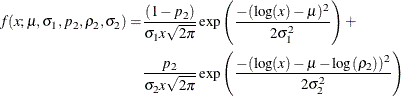You can verify thatserves as the log of the scale parameter(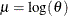). The following PROC FCMP steps encode this formulation in a distribution named SLOGNMIX2 for use with PROC SEVERITY:

/*- Define Mixture of 2 Lognormal Distributions with a Log-Scale Parameter -*/
proc fcmp library=sashelp.svrtdist outlib=work.sevexmpl.models;
function slognmix2_description() $128; return ("Mixture of two lognormals with a log-scale parameter Mu"); endsub; function slognmix2_scaletransform()$8;
return ("LOG");
endsub;

function slognmix2_pdf(x, Mu, Sigma1, p2, Rho2, Sigma2);
Mu1 = Mu;
Mu2 = Mu + log(Rho2);
pdf1 = logn_pdf(x, Mu1, Sigma1);
pdf2 = logn_pdf(x, Mu2, Sigma2);
return ((1-p2)*pdf1 + p2*pdf2);
endsub;

function slognmix2_cdf(x, Mu, Sigma1, p2, Rho2, Sigma2);
Mu1 = Mu;
Mu2 = Mu + log(Rho2);
cdf1 = logn_cdf(x, Mu1, Sigma1);
cdf2 = logn_cdf(x, Mu2, Sigma2);
return ((1-p2)*cdf1 + p2*cdf2);
endsub;

subroutine slognmix2_parminit(dim, x[*], nx[*], F[*], Ftype,
Mu, Sigma1, p2, Rho2, Sigma2);
outargs Mu, Sigma1, p2, Rho2, Sigma2;
array m / nosymbols;
p2 = 0.5;
Rho2 = 0.5;
median = svrtutil_percentile(0.5, dim, x, F, Ftype);
Mu = log(2*median/1.5);
call svrtutil_rawmoments(dim, x, nx, 1, m);
lm1 = log(m);

/* Search Rho2 that makes log(sample mean) > Mu */
do while (lm1 <= Mu and Rho2 < 1);
Rho2 = Rho2 + 0.01;
Mu = log(2*median/(1+Rho2));
end;
if (Rho2 >= 1) then
/* If Mu cannot be decreased enough to make it less
than log(sample mean), then revert to Rho2=0.5.
That will set Sigma1 and possibly Sigma2 to missing.
PROC SEVERITY replaces missing initial values with 0.001. */
Mu = log(2*median/1.5);

Sigma1 = sqrt(2.0*(log(m)-Mu));
Sigma2 = sqrt(2.0*(log(m)-Mu-log(Rho2)));
endsub;

subroutine slognmix2_lowerbounds(Mu, Sigma1, p2, Rho2, Sigma2);
outargs Mu, Sigma1, p2, Rho2, Sigma2;
Mu = .; /* Mu has no lower bound */
Sigma1 = 0; /* Sigma1 > 0 */
p2 = 0; /* p2 > 0 */
Rho2 = 0; /* Rho2 > 0 */
Sigma2 = 0; /* Sigma2 > 0 */
endsub;

subroutine slognmix2_upperbounds(Mu, Sigma1, p2, Rho2, Sigma2);
outargs Mu, Sigma1, p2, Rho2, Sigma2;
Mu = .; /* Mu has no upper bound */
Sigma1 = .; /* Sigma1 has no upper bound */
p2 = 1; /* p2 < 1 */
Rho2 = 1; /* Rho2 < 1 */
Sigma2 = .; /* Sigma2 has no upper bound */
endsub;
quit;


As shown in previous examples, an important aspect of defining a distribution for use with PROC SEVERITY is the definition of the PARMINIT subroutine that initializes the parameters. For mixture distributions, in general, the parameter initialization is a nontrivial task. For a two-component mixture, some simplifying assumptions make the problem easier to handle. For the initialization of SLOGNMIX2, the initial values of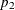and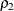are fixed at 0.5, and the following two simplifying assumptions are made:

• The median of the mixture is the average of the medians of the two components: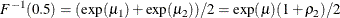Solution of this equation yields the value ofin terms ofand the sample median.

• Each component has the same mean, which implies the following: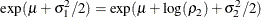If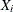represents the random variable of component distribution i and X represents the random variable of the mixture distribution, then the following equation holds for the raw moment of any order k: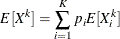This, in conjunction with the assumption on component means, leads to the equations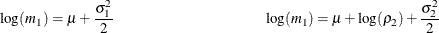where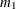denotes the first raw moment of the sample. Solving these equations leads to the following values of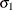and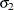: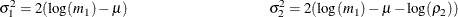Note thathas a valid value only if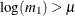. Among the many possible methods of ensuring this condition, the SLOGNMIX2_PARMINIT subroutine uses the method of doing a linear search over.

Even when the preceding assumptions are not true for a given problem, they produce reasonable initial values to help guide the nonlinear optimizer to an acceptable optimum if the mixture of two lognormal distributions is indeed a good fit for your input data. This is illustrated by the results of the following steps that fit the SLOGNMIX2 distribution to simulated data, which have different means for the two components (12.18 and 22.76, respectively), and the median of the sample (15.94) is not equal to the average of the medians of the two components (7.39 and 20.09, respectively):

/*-------- Simulate a lognormal mixture sample ----------*/
data testlognmix(keep=y);
call streaminit(12345);
Mu1 = 2;
Sigma1 = 1;
i = 0;
do j=1 to 2000;
y = exp(Mu1) * rand('LOGNORMAL')**Sigma1;
output;
end;
Mu2 = 3;
Sigma2 = 0.5;
do j=1 to 3000;
y = exp(Mu2) * rand('LOGNORMAL')**Sigma2;
output;
end;
run;

/*-- Fit and compare scale regression models with 2-component  --*/
/*-- lognormal mixture and the standard lognormal distribution --*/
options cmplib=(work.sevexmpl);

proc severity data=testlognmix print=all plots(histogram kernel)=all;
loss y;
dist slognmix2 logn;
run;



The comparison of the fit statistics of SLOGNMIX2 and LOGN, as shown in Output 23.7.1, confirms that the two-component mixture is certainly a better fit to these data than the single lognormal distribution.

Output 23.7.1: Comparison of Fitting One versus Two Lognormal Components to Mixture Data

All Fit Statistics
Distribution -2 Log
Likelihood
AIC AICC BIC KS AD CvM
slognmix2 38343 * 38353 * 38353 * 38386 * 0.52221 * 0.19843 * 0.02728 *
Logn 39073   39077   39077   39090   5.86522   66.93414   11.72703

The comparative plot of probability densities in Output 23.7.2 shows that the density function of the mixture distribution is bimodal. In fact, one of the key motivations for using mixture distributions is to find better-fitting models for multimodal data.

The P-P and Q-Q plots in Output 23.7.3 visually confirm that SLOGNMIX2 fits the data very well.

Output 23.7.2: Comparison of PDF Estimates of the Fitted Models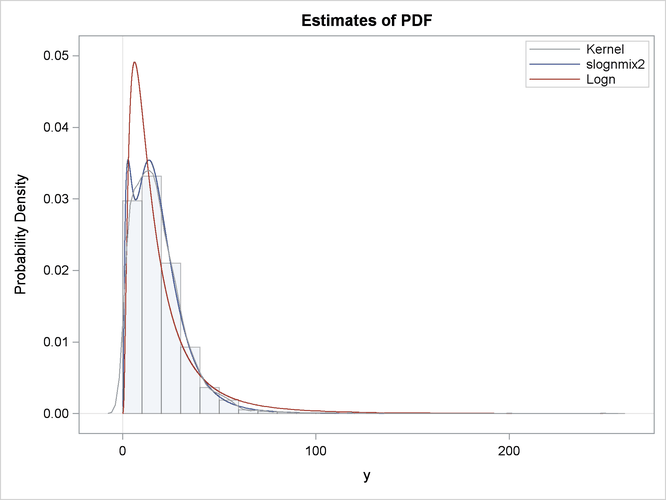Output 23.7.3: P-P and Q-Q Plots to Evaluate SLOGNMIX2 Fit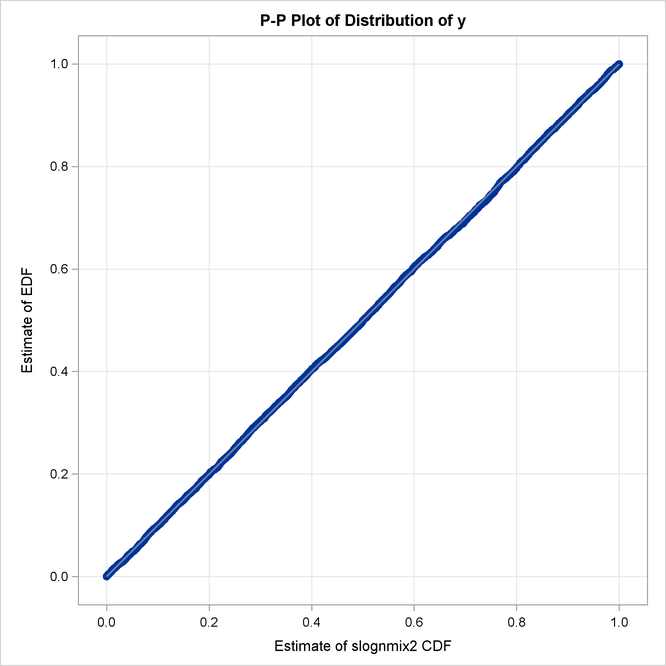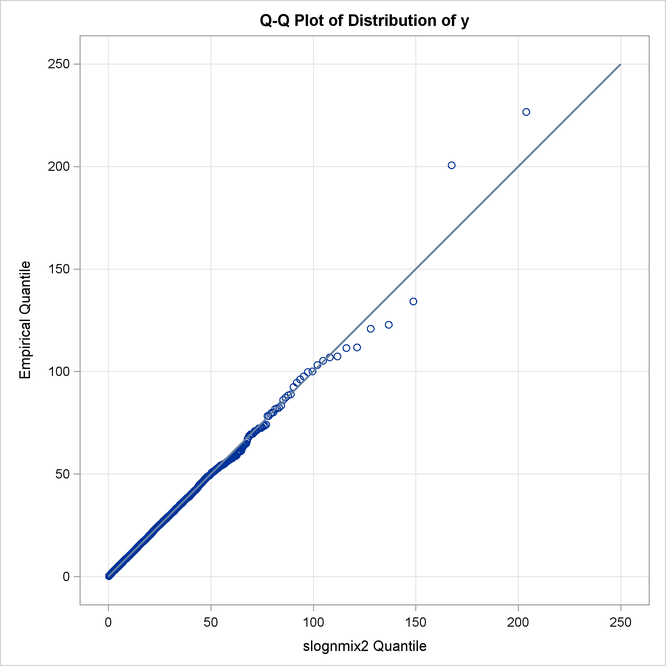The detailed results for the SLOGNMIX2 distribution are shown in Output 23.7.4. According to the "Initial Parameter Values and Bounds" table, the initial value ofis not 0.5, indicating that a linear search was conducted to ensure.

Output 23.7.4: Detailed Estimation Results for the SLOGNMIX2 Distribution

The SEVERITY Procedure
slognmix2 Distribution

Distribution Information
Name slognmix2
Description Mixture of two lognormals with a log-scale parameter Mu
Distribution Parameters 5

Initial Parameter Values and Bounds
Parameter Initial
Value
Lower
Bound
Upper
Bound
Mu 2.92006 -Infty Infty
Sigma1 0.10455 1.05367E-8 Infty
P2 0.50000 1.05367E-8 1.00000
Rho2 0.72000 1.05367E-8 1.00000
Sigma2 0.81728 1.05367E-8 Infty

Convergence Status
Convergence criterion (GCONV=1E-8) satisfied.

Optimization Summary
Optimization Technique Trust Region
Iterations 7
Function Calls 18
Log Likelihood -19171.5

Parameter Estimates
Parameter Estimate Standard
Error
t Value Approx
Pr > |t|
Mu 3.00922 0.01554 193.68 <.0001
Sigma1 0.49516 0.01451 34.13 <.0001
P2 0.40619 0.02600 15.62 <.0001
Rho2 0.37212 0.02038 18.26 <.0001
Sigma2 1.00019 0.02124 47.09 <.0001

By using the relationship that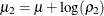, you can see that the final parameter estimates are indeed close to the true parameter values that were used to simulate the input sample.